# Working With Date and Time in Excel

Date and time in excel are treated a bit differently in excel than in other spreadsheets software. If you don't know how Excel date and time work, you may face unnecessary errors.

So, in this article, we will learn everything about the date and time of Excel. We will learn, what are dates in excel, how to add time in excel, how to format date and time in excel, what are date and time functions in excel, how to do date and time calculations (adding, subtracting, multiplying etc. with dates and times).

What is Date and Time in Excel?

Many of you may already know that Excel dates and time are nothing but serial numbers. A date is a whole number and time is a fractional number. Dates in excel have different regional formatting. For example, in my system, it is mm/dd/YYYY (we will use this format throughout the article). You may be using the same date format or you could be using dd/mm/YYYY date format.

Date Formatting of Cell

There are multiple options available to format a date in Excel. Select a cell that may contain a date and press CTRL+1. This will open the Format Cells dialogue box. Here you can see two formatting options as Date and Time. In these categories, there are multiple date formattings available to suit your requirements.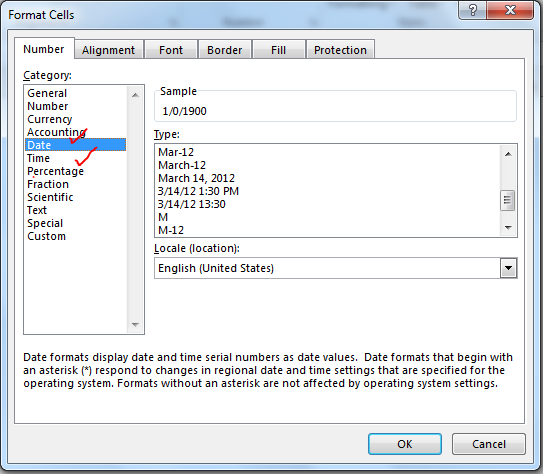Dates in Excel are mare serial numbers starting from 1-Jan-1900. A day in excel is equal to 1. Hence 1-Jan-1900 is 1, 2-Jan-1900 is 2, and 1-Jan-2000 is 36526.

Fun Fact: 1900 was not a leap year but excel accepts 29-Feb-1900 as a valid date. It was a desperate glitch to compete Lotus 1-2-3 back in those days.

Shortcut to enter static today's date in excel is CTRL+; (Semicolon).

To add or subtract a day from a date you just need to subtract or add that number of days to that date.

Time:
Excel by default follows the hh:mm format for time (0 to 23 format). The hours and minutes are separated by a colon without any spaces in between. You can change it to hh:mm AM/PM format. The AM/PM must have 1 space from the time value. To include seconds, you can add :ss to hh:mm (hh:mm:ss). Any other time format is invalid.

Time is always associated with a date. The date comes before the time value separated with a space from time. If you don't mention a date before time, by default it takes the first date of excel (which is 1/1/1900). Time in excel is a fractional number. It is shown on the right side of the decimal.

Hours:

Since 1 day is equal to 1 in excel and 1 day consists of 24 hours, 1 hour is equal to 1/24 in excel. What does that mean? It means that if you want to add or subtract 1 hour to time, you need to add or subtract 1/24. See the image below.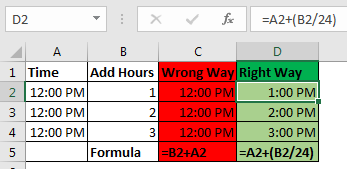you can say that 1 hour is equal to 0.041666667 (1/24).

Calculate hours between time in Excel

Minutes:

From the explanation of the hour in excel, you must have guessed that 1 Minute in excel is equal to 1/(24x60) (or 1/1440 or 0.000694444).

If you want to add a minute to an excel time, add 1/(24x60). See the image below. Sometimes you get the need to Calculate Minutes Between Dates & Time In Excel, you can read it here.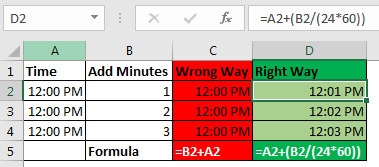Seconds:

Yes, a second in Excel is equal to 1/(24x60x60). To add or subtract seconds from a time, you just need to do the same things as we did in minutes and hours.

Date and Time in one cell

Dates and times are linked together. A date is always associated with a valid date and time is always associated with a valid excel date. Even if you are not able to see one of them.

If you only enter a time in a cell, the date of that cell will  1-Jan-1900, even if you are not able to see it. If you format that cell as a date-time format, you can see the associated date. Similarly, if you don't mention time with the date, by default 12:00 AM is attached. See the image below.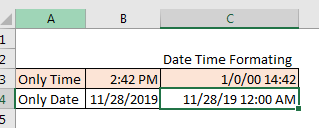In the image above, we have time only in B3 and date only in B4. When we format these cells as mm/dd/yy hh:mm, we get both, time and date in both cells.

So, while doing date and time calculations in excel, keep this in check.

No Negative Time

As I told you the date and time in excel starts from 1-Jan-1900 12:00 AM. Any time before this is not a valid date in excel. If you subtract a value from a date that leads to before 1-Jan-1900 12:00, even one second, excel will produce ###### error. I have talked about it here and in Convert Date and Time from GMT to CST. It happens when we try to subtract something that leads to before 1 Jan-1900 12:00. Try it yourself. Write 12:00 PM and subtract 13 hours from it. see what you get.

### Calculations with Dates and Time in Excel

Adding days to a date in excel is easy. To add a day to date just add 1 to it. See the image below.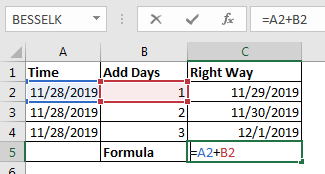You should not add two dates to get the future date, as it will sum up the serial numbers of those days and you may get a date far in the future.

Subtracting Days from Date:

If you want to get a backdate from a date a few days before, then just subtract that number of days from the date and you will get backdate. For example, if I want to know what date was before 56 days since TODAY then I would write this formula in the cell.

 =TODAY()-56

This will return us the date of 56 before the current date.
Note: Remember that you can not have a date before 1/Jan/1900 in excel. If you get ###### error, this could be the reason.

Days between two dates:
To calculate days between two dates we just need to subtract the start date from the end date. I have already done an article on this topic. Go and check it out here. You can also use the Excel DAYS Function to calculate days between a start date and end date.

There's been a lot of queries on how to add time to excel as many people get confusing results when they do it. There are two types of addition in times. One is adding time to another time. In this case, both times are formatted as hh:mm time format. In this case, you can simply add these times.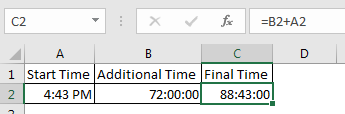The second case is when you don't have additional time in time format. You just have numbers of hours, minutes and seconds to add. In that case, you need to convert those numbers to their time equivalents. Note these points to add hours, minutes and seconds to a date/time.

• To add N hours to an X time use formula =X+(N/24) (As 1=24 hours)
• To Add N minutes to X time use formula = X+(N/(24*60))
• To Add N Second to X time use formula = X+(N/(24*60*60))

Subtracting Times

It's the same as adding time, just make sure that you don't end up with a negative time value when subtracting, because there is no such thing as a negative number in excel.

Note: When you add or subtract time in excel that exceeds 24 hours of difference, excel will roll to the next or previous date. For example, if you subtract 2 hours from 29-Jan-2019 1:00 AM then it will roll back to 28-Jan-2019 11:00 PM. If you subtract 2 hours from 1:00 AM (does not have the date mentioned), Excel will return ###### error. I have told the reason at the beginning of the article.
You can't just add multiples of 30 to add months to date as different months have a different number of days. You need to be careful while adding months to Date. To add months to a date in excel, we use EDATE function of excel. Here I have a separate article on adding months to a date in different scenarios.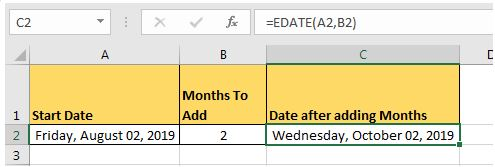Adding years to date:

Just like adding months to a date, it is not straightforward to add years to date. We need to use YEAR, MONTH, DAY function to add years to date. You can read about adding years to date here.

If you want to calculate years between dates then you can use this.

### Excel Date and Time Handling Functions:

Since date and time are special in Excel, Excel provides special functions to handle them. Here I am mentioning a few of them.

1. TODAY(): This function returns today's date dynamically.
2. DAY(): Returns Day of the month (returns number 1 to 31).
3. DAYS(): Used to count the number of days between two dates.
4. MONTH(): Used to get the month of the date (returns number 1 to 12).
5. YEAR(): Returns year of the date.
6. WEEKNUM(): Returns the weekly number of a date, in a year.
7. WEEKDAY(): Returns the day number in a week (1 to 7) of the supplied date.
8. WORKDAY():  Used to calculate working days.
9. TIMEVALUE(): Used to extract Time value (serial number) from a text formatted date and time.
10. DATEVALUE(): Used to extract date value (serial number) from a text formatted date and time.

These are some of the most useful data and time functions in excel. There are plenty more date and time functions. You can check them out here.

Date and Time Calculations

If I explain all of them here, this article will get too long. I have divided these time calculation techniques in excel into separate articles. Here I am mentioning them. You can click on them to read.

So yeah guys, this is all about the date and time in excel you need to know about. I hope this article was useful to you. If you have any queries or suggestions, write them down in the comments section below.

Terms and Conditions of use

The applications/code on this site are distributed as is and without warranties or liability. In no event shall the owner of the copyrights, or the authors of the applications/code be liable for any loss of profit, any problems or any damage resulting from the use or evaluation of the applications/code.